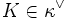# Supercharacter theory

## Definition

Let$G$ be a finite group and$F$ be a splitting field for$G$. A supercharacter theory of$G$ over$F$ is a partition$\kappa$ of the conjugacy classes of$G$ and a partition$\kappa^\vee$ of the irreducible characters of$G$ over a splitting field such that:

1. The identity element is its own block in$\kappa$
2.$|\kappa| = |\kappa^\vee|$
3. For each block$K \in \kappa^\vee$, there exists a corresponding character$\chi^K$ which is a positive integer combination of the characters in$K$ such that$\chi^K$ is constant on the blocks of$\kappa$.

### Terminology

Each block of conjugacy classes is termed a superconjugacy class, and the term may also be used for the union of all the conjugacy classes (i.e., the set of group elements).

Any positive integer combination that works for (3) is termed a supercharacter for this supercharacter theory. All supercharacters for a given block are scalar multiples of each other. We may distinguish the supercharacter that uses the smallest positive integer combination from the others.

## Examples

### Extreme cases

For every finite group, there are two extreme supercharacter theories. Further, unless the group is trivial or cyclic of order two, these are distinct from each other:

• The supercharacter theory where all the blocks are singleton subsets, i.e., each conjugacy class is its own block in$\kappa$ and each irreducible representation is its own block in$\kappa^\vee$.
• The supercharacter theory where the identity element is one block and all other conjugacy classes are the other block. On the representation side, the trivial representation forms one block and all other representations form another block.